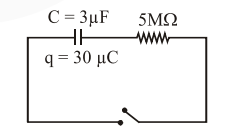# The circuit shown in the figure consists of`
Question:

The circuit shown in the figure consists of a charged capacitor of capacity $3 \mu \mathrm{F}$ and a charge of $30 \mu \mathrm{C}$. At time $\mathrm{t}=0$, when the key is closed, the value of current flowing through the $5 \mathrm{M} \Omega$ resistor is ' $x^{\prime} \mu-A$. The value of 'x to the nearest integer is_________.Solution:

$\mathrm{i}_{0}=\frac{\mathrm{V}}{\mathrm{R}}=\frac{30 / 3}{5 \times 10^{6}}=2 \times 10^{-6}$